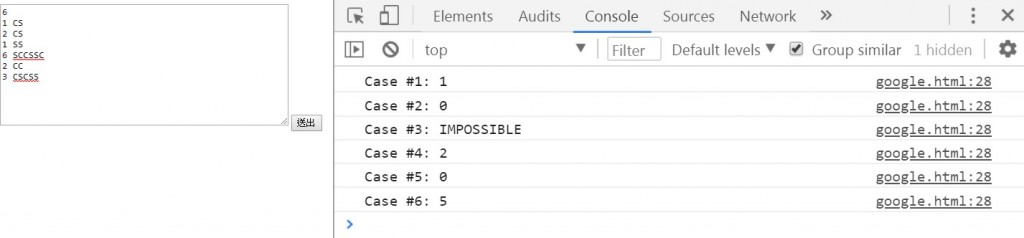#5

## [Google Code Jam][資格賽]Saving The Universe Again(再次拯救宇宙)

1.C：充電，將初始攻擊力1翻倍變為2
2.S：射擊，將造成目前攻擊力2點傷害
3.C：充電，將目前攻擊力2翻倍變為4
4.C：充電，將目前攻擊力4翻倍變為8
5.S：射擊，將造成目前攻擊力8點傷害

3
1 CS
2 CS
1 SS

Case #1: 1
Case #2: 0
Case #3: IMPOSSIBLE

``````<html>
<body>
<!--透過textarea輸入多行指令-->
<textarea id="input" rows="10" cols="50"></textarea>
<!--透過button執行事件-->
<input type="button" value="送出" onclick="send()"/>
<script>

function send(){
//取得攻擊指令
let word = document.getElementById('input').value;
//總共有幾行指令
let rowCount = word.split('\n').length-1;
//用來裝每次攻擊的結果
let arrResult = new Array();

//開始讀取指令(把盾的血量和攻擊指令傳到處理的函式)
for(let i=1;i<=rowCount;i++){
//取這一行的開始和最後位置
let first = findIndex(word,'\n',i);
let last = findIndex(word,'\n',i+1) || word.length;
//擷取那一行的字串，開始擷取的地方+1才不會截到斷行
let rowStr = word.slice(first+1,last);
//把那行的字串用空白分割開來，因為前面是血量，後面是指令
let hp = rowStr.split(' ');
let command = rowStr.split(' ');
//送去判斷能不能擋下這次攻擊，然後要交換幾次
arrResult[(i - 1)] = 'Case #' + i + ': ' + handleCommand(hp, command);
//印出結果
console.log('Case #' + i + ': ' + handleCommand(hp, command));
}
};

//處理指令
//hp:盾牌血量 command:攻擊指令
function handleCommand(hp, command) {
//先計算目前傷害值
let killValue = getKillValue(command);
//計算次數
let count = -1;
do {
if (count === -1) { //第一次先單純判斷「有沒有可能達成」或「有沒有需要換位置」
//如果他目前傷害值小於盾的血量就不需要換
if (Number(killValue) <= Number(hp)) {
return '0';
}
//如果他的S的數量大於盾的血量就沒不可能達成
if ((command.replace(/C/g, '')).length > Number(hp)) {
return 'IMPOSSIBLE';
}
count = 0; //下一次的迴圈就跑else
}
else { //第二次開始要換位置
count += 1; //先計算次數
//改變位置，讓他去跑看看
command = revisionCommand(command);
}
}
while (hp < getKillValue(command)); //如果總傷害量超過血量就繼續跑迴圈

//過了以後回傳次數
return count;

};

//計算傷害值
//command:指令
function getKillValue(command) {
//規則是 C:充電 S:射擊
let killValue = 0; //總傷害量
let basedKill = 1; //目前傷害量

for (let i = 0; i <= command.length - 1; i++) {
switch (command[i]) {
case "C": { //集氣
basedKill *= 2;
break;
};
case "S": { //加上目前傷害值
killValue += basedKill;
break;
};
};
};

return killValue;
};

//改變指令
//command:當前攻擊指令
function revisionCommand(command) {
//規則是用最少的移動來讓指令傷害不超過盾牌血量
//也就是說在C是充能和S是攻擊的狀況下，可以推出以下結論
//一、只要C後面沒有S就等同不會造成更高的傷害，所以就在每一次交換的時候把C往S後面移動
//二、由於要在最少次數達成，所以優先處理最後出現的CS，因為CS出現在越後面代表傷害越高

//尋找指令中最後出現的CS然後將他們換位置
let lastCS = command.lastIndexOf('CS');
//把指令放進陣列中
let arrCommand = command.split('');
//把原本位置中的C換成S，S換成C
arrCommand[lastCS] = 'S';
arrCommand[lastCS + 1] = 'C';

//把更改過位置的陣列轉成字串後回傳
return arrCommand.join('');
}

//尋找字串
//str:被尋找的字串  findStr:要尋找的字串  findNum:要找第幾個出現的字串
function findIndex(str, findStr, findNum) {
//先取得第一次出現的位置
let index = str.indexOf(findStr);

//之後去跑看要找第幾次出現的地方
for (let i = 0; i < findNum - 1; i++) {
//從上一次出現的地方開始找
index = str.indexOf(findStr, index + 1);
}

//如果是-1代表沒找到就回傳undefined
if (index === -1) {
index = undefined;
}

//回傳最後一次出現的位置
return index;
};
</script>

</body>
</html>
``````0